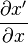# LSODE

NLA
QRSlv
CMSlv
IPSlv
NLP
CONOPT
IPOPT
TRON
MINOS
Opt
NGSlv
DAE/ODE
IDA
LSODE
DOPRI5
LA
Linsolqr
Linsol
LP
MakeMPS
Logic
LRSlv

This is the ASCEND binding to the well-known LSODE solver from LLNL. Provides multi-step ODE integration techniques both implicit and explicit (BDF and AM).

Note that although LSODE is an ODE solver, it is used in ASCEND as a DAE solver through the use of an 'inner loop' solver inside LSODE. The architecture at this stage is a bit messy: LSODE just wraps around the Solver instance of QRSlv currently assigned to the model (Simulation::setSolver). This needs to be generalised so that any NLA solver can be used for the inner loop.

LSODE extracts solutions for the differential variables using the QRSlv solution of from the inner loop (by FIXing the states and FREEing the derivatives). Then these derivatives and the corresponding differential variable are given to LSODE for time-stepping.

The use of an inner loop will make LSODE slower that IDA but it does have the benefit of giving a jacobian matrix from which system eigenvalues can be computed:$\frac{\partial x'}{\partial x}$

This matrix is accessible using the integrator_write_matrix or Integrator::writeMatrix function and is written in a form that can be read into the Python scipy tool, Matlab, and elsewhere (the MatrixMarket format).

## Solver parameters

meth
miter
maxord
timing
rtolvect
rtol
atolvect
atol